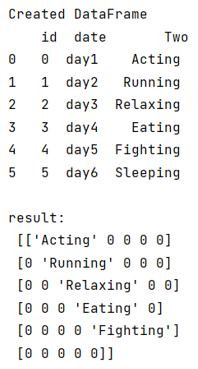# Transforming a DataFrame

In this article, we will learn how to transform a DataFrame so that we can convert the DataFrame values into matrix? By Pranit Sharma Last updated : September 25, 2023

Pandas is a special tool that allows us to perform complex manipulations of data effectively and efficiently. Inside pandas, we mostly deal with a dataset in the form of DataFrame. DataFrames are 2-dimensional data structures in pandas. DataFrames consist of rows, columns, and data.

## Problem statement

Sometimes, we need to transform a DataFrame so that we can understand the data patterns in a better way, sometimes we transform our DataFrame in such a way so that we convert all the values into a matrix. In this article, we are going to learn how to transform a DataFrame so that we can convert the DataFrame values into the matrix.

## Transforming a DataFrame

For this purpose, we will use pandas.DataFrame.pivot() method. This method is used to reshape the given DataFrame according to index and column values. It is used when we have multiple items in a column, we can reshape the DataFrame in such a way that all the multiple values fall under one single index or row, similarly, we can convert these multiple values as columns.

Syntax of the pivot() method is:

```DataFrame.pivot(
index=None,
columns=None,
values=None
)
```
Note

To work with pandas, we need to import pandas package first, below is the syntax:

```import pandas as pd
```

Let us understand with the help of an example,

## Python program for transforming a DataFrame

```# Importing pandas package
import pandas as pd

# Creating a Dictionary
d = {
'id':[0,1,2,3,4,5],
'date':['day1','day2','day3','day4','day5','day6'],
'Two':['Acting','Running','Relaxing','Eating','Fighting','Sleeping']
}

# Creating a DataFrame
df = pd.DataFrame(d)

# Display DataFrame
print("Created DataFrame\n",df,"\n")

# Pivot dataframe
result = df.pivot(*df).reindex(['day1', 'day2', 'day3', 'day4', 'day5'], axis=1).fillna(0).values

# Display result
print("result:\n",result)
```

### Output

The output of the above program is: